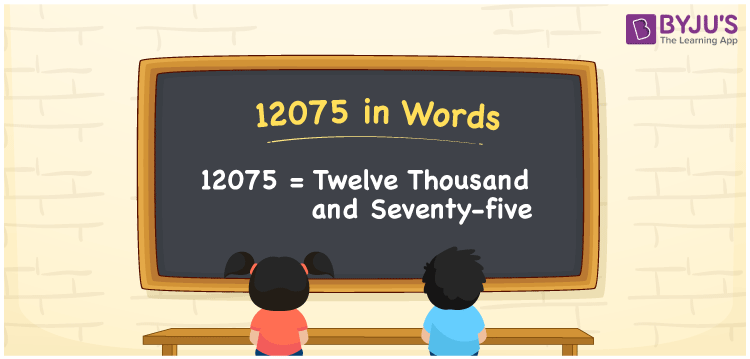# 12075 in Words

We can write the number 12075 in words as Twelve Thousand and Seventy-Five. For example, if you bought a diamond nose pin worth Rs. 12075, then you can say, “I bought a diamond nose pin worth Rupees Twelve Thousand and Seventy-Five”. The number 12075 is a cardinal number since it conveys a specific quantity. Learn the technique of converting the number 12075 into words here.

 12075 in Words Twelve Thousand and Seventy-Five Twelve Thousand and Seventy-Five in numerical form 12075

## 12075 in English Words

Generally, we write the numbers in words with the help of the English alphabet. Therefore, we can write the number 12075 in words as Twelve Thousand and Seventy-Five.## How to Write 12075 in Words?

The number 12075 is a five-digit number. So, we need a place value table of 5 columns. The below table is the place value chart for the number 12075.

 Ten Thousand Thousand Hundreds Tens Ones 1 2 0 7 5

Therefore, we can write the expanded form as:

1 x Ten Thousand + 2 x Thousand + 0 x Hundred + 7 x Ten + 5 x One

= 1 x 10000 + 2 x 1000 + 0 x 100 + 7 x 10 + 5 x 1

= 10000 + 2000 + 0 + 70 + 5

= 10000 + 2000 + 70 + 5

= 12075

= Twelve Thousand and Seventy-Five

Hence, 12075 in words is written as Twelve Thousand and Seventy-Five

Interesting way of writing 12075 in words

1 = One

12 = Twelve

120 = One Hundred and Twenty

1207 = One Thousand Two Hundred Seven

12075 = Twelve Thousand and Seventy-Five

Thus, the word form of the number 12075 is Twelve Thousand and Seventy-Five

12075 is a natural number that is the successor of 12074 and the predecessor of 12076

• 12075 in words – Twelve Thousand and Seventy-Five
• Is 12075 an odd number? – Yes
• Is 12075 an even number? – No
• Is 12075 a perfect square number? – No
• Is 12075 a perfect cube number? – No
• Is 12075 a prime number? – No
• Is 12075 a composite number? – Yes

## Frequently Asked Questions on 12075 in Words

Q1

### Write 12075 in words.

12075 in words is written as Twelve Thousand and Seventy-Five.
Q2

### What is the value of 10000 + 2075 in words?

10000 + 2075 = 12075 i.e., Twelve Thousand and Seventy-Five.
Q3

### Is 12075 an odd number?

Yes, 12075 is an odd number.